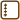A particle with given mass m is located somewhere on the line segment with given length L.

a) Estimate the smallest possible kinetic energy of the particle with the use of the principle of uncertainty.

b) Calculate this energy for the case of a bead with the mass of 1 g which is restricted to the string with the length of 10 cm.

c) Calculate this energy for the case of an electron on a line segment with the length 0.1 nm long, which is the typical size of an atom.

Keep in mind that this is only a rough estimate! We could obtain the precise value from the solution of the Schrödinger’s equation. We can consider the particle moving on the line segment with length L as particle in an infinitely deep potential well with the width L which cannot pass the borders of this well. Similar solved problems can be found in the section Infinitely deep potential well.

• #### Hint

Which principle of uncertainty restricts the determination of particle’s location?

What is the relation between the kinetic energy and the momentum of the particle?

• #### Solution

a) a) The minimum kinetic energy can be found as

$E_{\mbox{min}}=\frac{1}{2m}\langle p^2 \rangle_{\mbox{min}} .$

For uncertainty of the momentum Δp we have

$(\Delta p)^2=\langle p^2 \rangle\,-\,\langle p \rangle^2 .$

Since the line segment is not moving and the particle can freely move from left to right on the segment the expected value of its momentum is zero, i.e. $$\langle p \rangle = 0$$ and we can express

$\langle p^2 \rangle = (\Delta p)^2\,+\,\langle p \rangle^2=(\Delta p)^2\,+\,0=(\Delta p)^2 .$

Similarly for the uncertainty of the location Δx we can express

$(\Delta x)^2=\langle x^2 \rangle\,-\,\langle x \rangle^2 .$

If we place the well between points x = −L/2 and x = L/2 the problem is symmetric and the expected value of the particle’s location is zero, i.e. <x> = 0 and we can express

$(\Delta x)^2=\langle x^2 \rangle\,-\,0=\langle x^2 \rangle ,$ $(\Delta x)^2=\frac{1}{L}\int_{-L/2}^{L/2}x^2\,\mbox{d}x ,$ $(\Delta x)^2=\frac{1}{L}\,\left[\frac{x^3}{3}\right]_{-L/2}^{L/2} ,$ $(\Delta x)^2 = \frac{L^2}{12} .$

From the formula for principle of uncertainty

$(\Delta x)^2\,\cdot\,(\Delta p)^2 \ge \frac{\hbar^2}{4}$

We can derive that

$(\Delta p)^2 \ge \frac{\hbar^2}{4}\,\frac{1}{(\Delta x)^2} ,$ $(\Delta p)^2 \ge \frac{\hbar^2}{4}\,\frac{12}{L^2} ,$ $(\Delta p)^2 \ge \frac{3\hbar^2}{L^2} .$

The minimum kinetic energy of the particle is equal to

$E_{\mbox{min}}=\frac{1}{2m}\langle p^2\rangle=\frac{3\hbar^2}{2mL^2} .$

It is necessary to keep in mind that it is still a rough estimate. The precise value of the minimum energy can be calculated through Schrödinger’s equation as the smallest eigenvalue of the Hamiltonian for the specific situation. (It is solved in detail in the problem Symetrická nekonečná pravoúhlá jáma.)

If we stick to the rough estimate which comes from the principle of uncertainty we obtain these energies after the substitution of given values

b) $E_{\mbox{min}}=\frac{3\,\cdot\,\left(1.055{\cdot}10^{-34}\,\mbox{J s}\right)^2}{2\,\cdot\,10^{-3}\,\mbox{kg}\,\cdot\,\left(0.1\,\mbox{m}\right)^2}\,\dot{=}\,1.67{\cdot}10^{-63}\ \mbox{J}\,\dot{=}\,1.04{\cdot}10^{-44}\ \mbox{eV} ,$

c) $E_{\mbox{min}}=\frac{3\,\cdot\,\left(1.055{\cdot}10^{-34}\,\mbox{J s}\right)^2}{2\,\cdot\,9.11{\cdot}10^{-31}\,\mbox{kg}\,\cdot\,\left(10^{-10}\,\mbox{m}\right)^2}\,\dot{=}\,1.83{\cdot}10^{-18}\ \mbox{J}\,\dot{=}\,11.4\ \mbox{eV} .$

a) The energy of the particle with the mass m located somewhere on the line segment with given length L is equal to at least $\,\frac{3\hbar^2}{2mL^2} .$

b) The energy of the bead with the mass 1 g which is obliged to the string with the length 10 cm is equal to at least 1.04·10−44 eV. Motion with that kinetic energy is not really observable.

c) c) The energy of the electron on the line segment with given length 0.1 nm is equal to at least 11.4 eV.

• #### Comment

The minimum energy which we obtain from Schrödinger’s equation for the particle with the mass m in an infinitely deep perpendicular potential well of width L is equal to

$E_1=\frac{\hbar^2 \pi^2}{2mL^2} .$

(Detailed derivation can be found in Symetrická nekonečná pravoúhlá jáma.)

It is $$\frac{\pi^2}{3}\,\dot{=}\,3.3$$ times higher than the estimation we made from the principle of uncertainty.